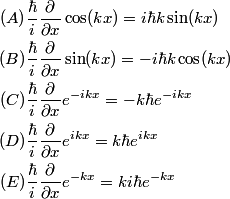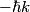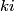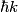## Solution to 1986 Problem 57

 We apply the linear momentum operator to each of the given functions:\begin{align*}(A)& \frac{\hbar}{i} \frac{\partial}{\partial x} \cos(k x) = i \hbar k \sin (k x) \\(B)& \frac{\hbar}{i...So, (A) and (B) are not even eigenfunctions of the linear momentum operator. (C), (D), and (E) are all eigenfunctions of the linear momentum operator, but the eigenvalue of (C) is$- \hbar k$ and the eigenvalue of (E) is$k i$. The eigenvalue of (D) is$\hbar k$, so the correct answer is (D).